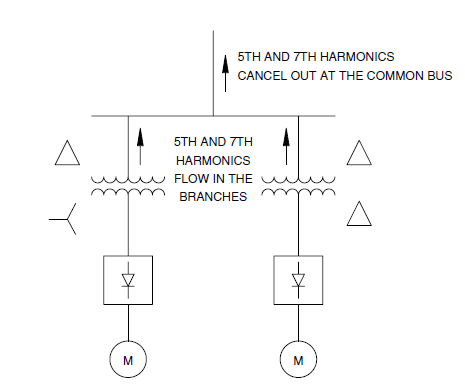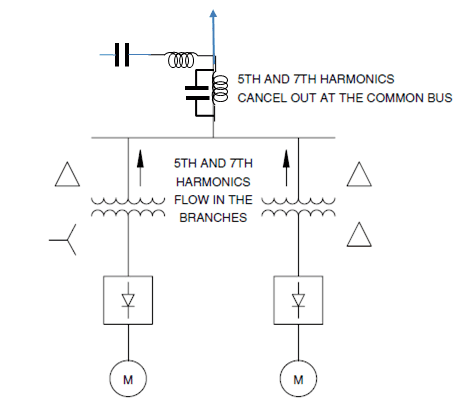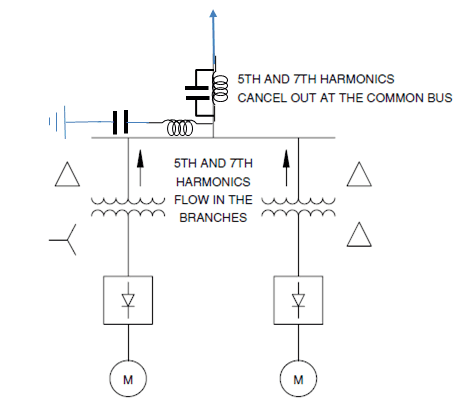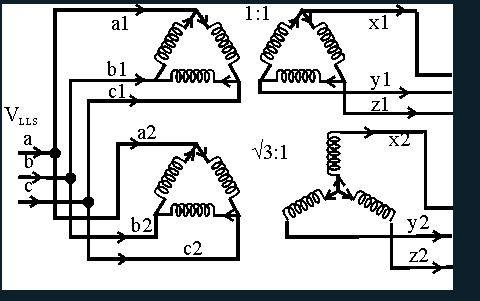# 5th and 7th Harmonic mitigation (D-yd transformer)

Hi,
I do not see how this method (illustrated in the figure below), can completely remove the 5th and 7th harmonic?

I know that in a wye-delta connection a 30 degree phase shift is introduced. But to fully remove the 5th harmonic we need a phase shift of 180/5 = 36, and for the 7th harmonic we need 180/7 = 25.71.

Since 36 and 25.71 degree is Close to 30 degree I assume they get greatly reduced. So parhaps they should not write "cancel out at the common bus" to avoid any confusion?Last edited:

In my opinion, it is difficult to mitigate the harmonics by changing phase since the wave will remain unchanged. In order to mitigate a harmonic one has to filter the harmonic by increase the series impedance and to reduce the shunt impedance in order to purge the harmonic.

The impedance depends on harmonic frequency-the resistance less due to skin effect and proximity-effect, but the reactance is direct proportional with frequency. A suitable capacitance parallel will form inductance together a harmonic barrier and in a series a shunt.

Cap=1/(2*pi()*f)/XL f=[5 or 7]*[50 or 60] XL=inductive reactance [ohm]
See[for instance]:
http://www.apqpower.com/assets/files/AReviewOfHarmMitigTech.pdfSorry. Wrong position of the shunt.But im just curious when the Author of the book (Power Quality by C. Sankaran) says that by shifting one load 30 degree with respect to the other, we can cancel out the 5th and 7th harmonic, assuming the loads are equal etc.

But we can not cancel them completely?

Lets say we have to 5th harmonic loads connected in parallel, and they are shifted 180/5=36 degrees, if they are shifted 36 degrees with respect to each other they would completely cancel if they were identical, correct?

However, if we only shift 30 degrees, we can not completely cancel them out, even if they are identical. But 30 degrees is kind of Close to 36 degrees, so we can some cancellation, and that is what the Author means when he says that 5th and 7th harmonic cancel at common bus, correct?

I found the "Power Quality of Mr. C.Sankaran" and indeed on the ch.4.10 HARMONIC CURRENT MITIGATION I read this sentence:

"As the result of this, the source does not see any significant amount of the 5th and 7th harmonics."

I split the 5 and 7 harmonic current wave in 10 equal parts duration and I use this formula[if the currents are equal]:

Sum[ two currents at time t]= SIN(h*w*t)+SIN(h*(w*t-fi)) where:

w=2*pi()*f[50 or 60]; h=5 or 7;t=n/f/h/10 n=number of time part [0 to 10].

fi=angle difference between currents.

In this way calculated for fi=0 [both current on the same phase-respectively-] Sum [rms]=1.818

If the currents are 30deg apart then Sum decrease to 0.1445 [7.9% from the initial]. It is not significant, may be.

By-the-way at 25.7 deg. for h=7 and 35 deg.for h=5 the Sum is 0 indeed.

•BjornFanden
Thank you for your time :)

Sorry. I forgot to extract the root. Then that has to be:

In this way calculated for fi=0 [both current on the same phase-respectively-] Sum [rms]=1.4142

If the currents are 30deg apart then Sum decrease to 0.3987 [28.19% from the initial]. It is significant, in my opinion.

Hi, I think that full cancellation is theoretical possible if the loads are equal, here's why:
In the delta-delta the currents are the same at primary and secondary.
$a1 = x1 = sin(5 \omega t)\\b1 = y1 = sin(5 \omega t - 120^{\circ})\\c1 = z1 = sin(5 \omega t + 120^{\circ})$
for the delta-wye we have on the secondary side:
$x2 = sin(5 \omega t+30^{\circ}\times 5) = sin(5 \omega t+150^{\circ})\\ y2 = sin(5 \omega t - 120^{\circ}\times 5 +30^{\circ}\times 5) = sin(5 \omega t -90^{\circ})\\ z2= sin(5 \omega t + 120^{\circ}\times 5 +30^{\circ}\times 5) = sin(5 \omega t +30^{\circ})$

Going from secondary to primary we have to include the $\sqrt3:1$ ratio
$A2= (i_{a}-i_{b})/\sqrt3 = (sin(5\omega t+150^{\circ})-sin(5\omega t +30^{\circ}))/\sqrt3 = -sin(5 \omega t)$

Similar can be shown for b2 and c2 that they are equal to b1 and c1, respectively (for 5th harmonic).

Can someone verify my calculations, so we do not cause any problems for other wondering about the same.Bump, could any kind soul check if my last post (#8) is correct so we can settle for an answer.

Bump, ref. post #8.

In my opinion, since the apparent power on low-voltage side is equal to apparent power at high-voltage side and the line-to-line voltage is the same the line current has to be the same. So no correction of sqrt(3) is required.
However, the winding turns ratio has to be w1/w2=sqrt(3).
For other explanations see your new post of Dec.2